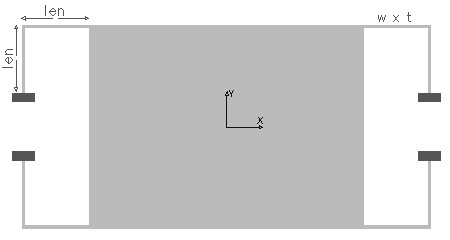BENTBEAM,len,w,t,ymod,pois,sel
Design of a corner beam or bent beam suspension

len         length of one leg of the bent beam in µm
w           width of the beam in µm
t            thickness of the beam in µm
ymod    Young's modulus of the material of the beam in GPa
pois       Poisson's ratio
sel         number denoting the selected result.
Use 1 for spring constant in X axis and 2 for spring constant in Y axis and 3 for spring constant in Z axisNotes

The bent beam is formed by two spring segments perpendicular to each other, joined at the corner of the central mass as shown in the picture. The two segments of the beam can have different length, but is assumed to be equal. The mass is suspended on four such bent beams. In the analysis only a single beam is considered, and depending on the number of bend beams used in the suspension the effective spring constant can be calculated.

If the spring constant of one beam is k1 and the second spring is k2, and if they are connected in parallel, the effective spring constant

Kparallel = k1 + k2.

If the two springs are connected in series the effective spring constant is

1/Kseries  = 1/k1 + 1/k2

The suspension allows the in-plane movement of the mass in X and in Y direction. It also has an out-of-plane movement perpendicular to the plane of the mass in Z direction. The stiffness of this suspension in all the three axis directions can be found out using this design interface. Since the beam segments have equal length and cross-section, the stiffness in X and Y axis are the same.

The X-Y plot shows the variation of in-plane stiffness (stiffness in X and Y) with the ratio of length to width (len / w) of the beam when the thickness is held constant at the given value. The 2D and 3D surface plot shows the out-of-plane deflection of the bent beam suspension.Assumptions

-The default material is Silicon with a Young's modulus of 180GPa and Poisson's ratio of 0.3.
-The beam has uniform rectangular cross section.
-The two segments of the beam are equal in length.
-Only one beam is considered in the analysis.
-In single beam analysis, the point where the mass is attached is assumed to be free to move.
-Only bending effects are considered. Axial deformations are assumed to be small compared to bending effects.